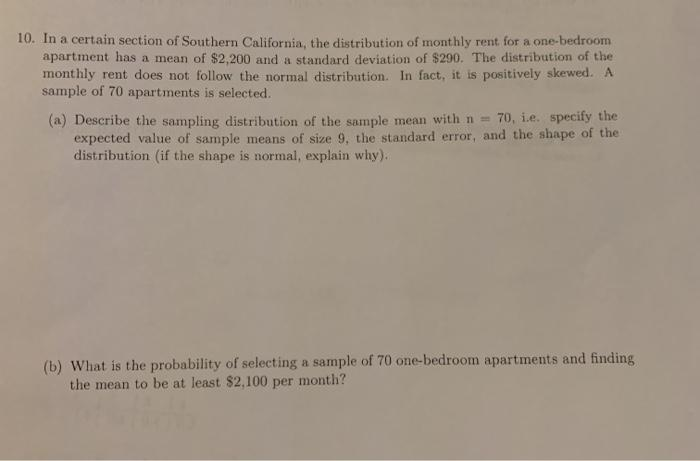# Question 10. In a certain section of Southern California, the distribution of monthly rent for a one-bedroom apartment has a mean of $2,200 and a standard deviation of$290. The distribution of the monthly rent does not follow the normal distribution. In fact, it is positively skewed. A sample of 70 apartments is selected. (a) Describe the sampling distribution of the sample mean with n = 70, i.e. specify the expected value of sample means of size 9, the standard error, and the shape of the distribution (if the shape is normal, explain why). (b) What is the probability of selecting a sample of 70 one-bedroom apartments and finding the mean to be at least $2,100 per month?EQGX2E The Asker · Probability and StatisticsTranscribed Image Text: 10. In a certain section of Southern California, the distribution of monthly rent for a one-bedroom apartment has a mean of$2,200 and a standard deviation of $290. The distribution of the monthly rent does not follow the normal distribution. In fact, it is positively skewed. A sample of 70 apartments is selected. (a) Describe the sampling distribution of the sample mean with n = 70, i.e. specify the expected value of sample means of size 9, the standard error, and the shape of the distribution (if the shape is normal, explain why). (b) What is the probability of selecting a sample of 70 one-bedroom apartments and finding the mean to be at least$2,100 per month?
More
Transcribed Image Text: 10. In a certain section of Southern California, the distribution of monthly rent for a one-bedroom apartment has a mean of $2,200 and a standard deviation of$290. The distribution of the monthly rent does not follow the normal distribution. In fact, it is positively skewed. A sample of 70 apartments is selected. (a) Describe the sampling distribution of the sample mean with n = 70, i.e. specify the expected value of sample means of size 9, the standard error, and the shape of the distribution (if the shape is normal, explain why). (b) What is the probability of selecting a sample of 70 one-bedroom apartments and finding the mean to be at least \$2,100 per month?